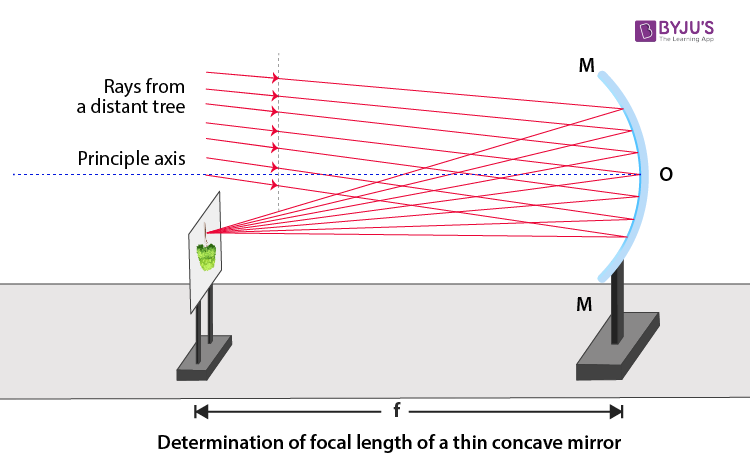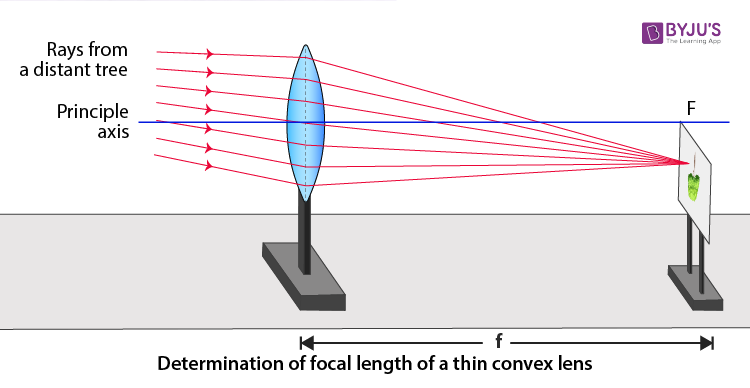Checkout JEE MAINS 2022 Question Paper Analysis : Checkout JEE MAINS 2022 Question Paper Analysis :

# Determination Of Focal Length Of Concave Mirror And Convex Lens

Before getting started with the experiment, it is important to understand a few terms that are used in a concave mirror and convex lens:

• Centre of curvature: For a curved mirror, the centre of curvature, C can be defined as the centre of a hollow glass sphere of which a curved mirror is a part.
• Radius of curvature: For a curved mirror, the radius of curvature, R can be defined as the radius of the hollow glass sphere of a spherical mirror is part.
• Principal axis: Principal axis is defined as the imaginary line passing through the pole and centre of curvature of the spherical mirror.
• Principal focus: Principal focus is defined as a point at which the reflected rays meet or appear to meet for the spherical mirror. For a concave mirror, the principal focus is in the front and for a convex mirror, the principal focus is at the behind.

Below is an experiment to determine the focal length of a concave mirror and convex lens.

## Aim

To determine the focal length of:

• Concave mirror
• Convex lens by obtaining the image of a distant object.

## Theory

### How to find focal length of concave mirror?

Following are the ways to obtain the focal length of the concave mirror:

• A concave mirror is defined as the spherical mirror whose reflecting surface is curved inwards and follows laws of reflection of light.
• The light rays coming from a distant object can be considered to be parallel to each other.
• If the image formed is real, inverted and very small in size, then the parallel rays of light meet the point in the front of the mirror.
• The image formed by the convex lens is real and can be obtained on the screen.
• f is used to denote the difference between the principal axis P and the focus F of the concave mirror.

### How to find the focal length of convex lens?

Following are the ways to obtain the focal length of convex lens:

• The convex lens is thicker in the middle and thinner at the edges and is also known as the converging lens.
• The refracted rays from the parallel beam of light converge on the other side of the convex lens.
• If the image is obtained at the focus of the lens, the image would be real, inverted and very small.
• f is the focal length which is the difference between the optical centre of the lens and the principal focus.
• The image can be obtained on the screen as the image formed by the lens is real.

## To determine focal length of a given concave mirror

### Materials Required

1. A concave mirror
2. A measuring scale
3. A screen holder
4. A mirror holder
5. A mirror stand

### Procedure

1. The distance between the selected distinct object should be more than 50 ft.
2. The concave mirror placed on the mirror stand and the distant object should be facing each other.
3. The screen should be placed in front of the reflecting surface of the mirror. To obtain a clear, sharp image the screen should be adjusted.
4. Using a metre scale the distance between the concave mirror and screen can be determined. The distance is the same as the focal length of the given concave mirror.
5. Repeat the above procedure thrice to calculate the average focal length.

### Experimental Setup### Observation Table

 Sl.no Position of concave mirror (M) Position of screen (S) Focal length = (M-S) cm 1 60 cm 50 cm 10 cm 2 60 cm 50 cm 10 cm 3 60 cm 50 cm 10 cm

### Calculation

Following is the mean value of the focal length of concave mirror:

 $$\begin{array}{l}\frac{f_{1}+f_{2}+f_{3}}{3}\;cm=10\;cm\end{array}$$

### Result

10 cm is the focal length of the concave mirror.

### Precautions

1. To get a well illuminated and distinct image of the distinct object, the distant object should be well illuminated.
2. A concave mirror should be always placed near an open window.
3. The polished surface of the concave mirror and the distinct object should be facing each other.
4. There should not be any hurdle between the rays of light from the object and the concave mirror.
5. The screen and the concave mirror stand should be parallel to the measuring scale.
6. The mirror holder along with the mirror should be perpendicular to the measuring scale.

## To determine focal length of a convex lens

### Materials Required

1. A wooden bench
2. A convex lens
3. A lens holder
4. A screen fixed to a stand
5. A measuring scale

### Experimental Setup### Procedure

1. Without disturbing the lens and screen, arrange both of them on the wooden bench.
2. Place the lens on the holder facing a distant object.
3. Place the holder with the screen on the bench.
4. The position of the screen should be such that the sharp image of the distant object is obtained on it.
5. The difference between the position of the lens and the screen is equal to the focal length of the given convex lens.
6. Now shift the focus to towards various other distant object and calculate the focal length of the convex lens.

### Observation Table

 Sl.no Position of convex lens (L) Position of screen (S) Focal length = (L-S) cm 1 60 cm 50 cm f1 = 10cm 2 60 cm 50 cm f2 = 10cm 3 60 cm 50 cm f3 = 10cm

### Calculation

Following is the mean value of the focal length of convex lens:

 $$\begin{array}{l}\frac{f_{1}+f_{2}+f_{3}}{3}\;cm=10\;cm\end{array}$$

### Result

10 cm is the focal length of the convex lens.

### Precautions

1. The placing of the convex lens should be vertical.
2. There should not be any hurdle between the rays of light from the object and the convex lens.
3. To get a well illuminated and distinct image of the distinct object, the distant object should be well illuminated.
4. The convex lens stand and the screen should be parallel to the measuring scale.

## Viva Questions

Q1. Differentiate between convex lens and concave lens.
Ans: Following is the table explaining the difference between the convex lens and the concave lens:

 Convex lens Concave lens The focal length is positive The focal length is negative Image obtained can be either real or virtual Image obtained is always virtual The light rays are converged towards the principal axis The light rays diverge away from the principal axis

Q2. Name two types of spherical mirrors
Ans: Following are the two types of spherical mirror:

• Concave mirror
• Convex mirror

Q3. What is the relationship between focal length T and the radius of curvature R of a concave mirror?
Ans: Following is the relationship between focal length and the radius of curvature:

 $$\begin{array}{l}f=\frac{R}{2}\end{array}$$

Q4. What is the mirror formula?
Ans: Following is the mirror formula:

 $$\begin{array}{l}\frac{1}{f}=\frac{1}{v}+\frac{1}{u}\end{array}$$

Q5. What is law of reflection for a mirror?
Ans: Following is the law of reflection for a mirror:

• The incident ray, the reflected ray and the normal all lie in the same plane.
• The angle of incidence is equal to the angle of reflection.

Test your knowledge on Focal length determination of concave mirror and convex lens

USEFUL INFO THANK YOU

2. Abhiram